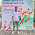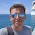# String Rotation in Java - Write a Program to check if strings are rotations of each other or not

String based algorithmic questions are very popular in Java interviews e.g. checking if two String is the anagram of each other (see), or checking if given String is a palindrome (see), or just finding permutations of given String (see). One of such popular String based interview questions is about to check if two Strings are a rotation of each other in Java. In order to solve this question, you should know what is String rotation? Well, A string is made of characters and you just rotate the String around any character e.g. "programming" will become "ingprogramm" if we rotate the String on trailing character 3 times. A k-rotation on a string takes the trailing k characters of the string and attaches it to the beginning of the string in the same order. You can rotate the String either in the clock wise (right from the top) or anti-clockwise(left from the top). The string can also be rotated in one go e.g. "123456" will become "456123" if we rotate 456 to the left around character "3".

Problem:
Write a program to check if two given String s1 and s2 are rotations of another. For example if s1 = "IndiaUSAEngland" and s2= "USAEnglandIndia" then your program should return true but if s2="IndiaEnglandUSA" then it should return false.

For the purpose of this program, you can assume that Strings are rotated on the right side, but you should ask this question to your interviewer before jumping into the solution. Paying attention to such details score brownie points on real Interview. Remember, attention to details is one of the desired quality for software engineers.

Solution:
The simplest solution of this complex problem is to concatenate the String with itself and check if the given rotation exists in this concatenated String. If it exists then the second string is a rotation of the first string.

Btw, before concatenating String, you should also first check the length of the two String. If they are of different length than two strings are definitely not the rotation of each other, but if they have the same length then you need to check further. This will result in faster solution because checking length is faster than checking if a substring exists in the given String.

Here is the exact algorithm to check if One String is a rotation of another:

1) check length of two strings, if length is not same then return false
2) concatenate given string to itself
3) check if rotated version of String exists in this concatenated string
4) if yes, then second String is rotated version of first string

Java Program to check if One String Rotation of Another
```import java.util.Scanner;

/*
* Java Program to check if one String is rotation of
* another.
*/
public class Main {

public static void main(String[] args) throws Exception {

Scanner scnr = new Scanner(System.in);
String input = scnr.nextLine();

String rotation = scnr.nextLine();

if (checkRotatation(input, rotation)) {
System.out.println(input + " and " + rotation
+ " are rotation of each other");
} else {
System.out.println("Sorry, they are not rotation of another");
}

scnr.close();
}

/**
* This method check is given strings are rotation of each other
* @param original
* @param rotation
* @return true or false
*/
public static boolean checkRotatation(String original, String rotation) {
if (original.length() != rotation.length()) {
return false;
}

String concatenated = original + original;

if (concatenated.indexOf(rotation) != -1) {
return true;
}

return false;
}
}

Output
IndiaVsAustralia
AustraliaVsIndia
Sorry, they are not rotation of another

IndiaVsEngland
EnglandIndiaVs
IndiaVsEngland and EnglandIndiaVs are rotation of each other```

You can see that when a user enters "IndiaVsAustralia" and "AustraliaVsIndia" then our program return false because they are not a rotation of each other, but, when the user enters "IndiaVsEngland" and "EnglandIndiaVs" then our program returns true because here two strings are the rotation of each other.

That's all about how to check if one String is a rotation of another in Java. As I said, the simplest way to solve this problem is to concatenate String with itself and check if rotation exists in the concatenated String or not. If it exists, it means they are a rotation of each other. If not, the first string is not a rotation of other.

Though, there are a couple of variant of this program which many interviewers ask as follow-ups e.g. how do you solve the problem if strings are rotated on the left side, or can you check if two strings are the rotation of another without using String concatenation. You can try solving those versions by yourself, but if you want to look for a solution, you can check it here.

Further Learning
The Coding Interview Bootcamp: Algorithms + Data Structures
Data Structures and Algorithms: Deep Dive Using Java
Algorithms and Data Structures - Part 1 and 2

Related String based Algorithmic Questions from Interviews, you may like to practice:
• How to Print duplicate characters from String? (solution)
• How to find duplicate characters in a String? (solution)
• How to check if String is Palindrome?(solution)
• How to return highest occurred character in a String? (solution)
• How to check if a String contains only digits?  (solution)
• How to reverse String in Java using Iteration and Recursion? (solution)
• How to count the number of vowels and consonants in a String? (solution)
• How to program to print first non-repeated character from String? (solution)
• How to count the occurrence of a given character in String? (solution)
• How to convert numeric String to an int? (solution)
• How to reverse words in a sentence without using library method? (solution)
• How to reverse a String in place in Java? (solution)

1./**
* Author : Anil Churasiya
* email : achaurasiya59@gmail.com
* Date : October 12, 2018
*/
import java.util.Scanner;

class StringRotation{
public static void main(String[] args) {
Scanner sc = new Scanner(System.in);
String str1 = sc.nextLine();
String str2 = sc.nextLine();
boolean status = isRotated(str1, str2);
if(status){
System.out.println("True");
}
else{
System.out.println("False");
}
}

public static boolean isRotated(String str1, String str2){
boolean status = false;
char arrStr1[] = str1.toCharArray();
char arrStr2[] = str2.toCharArray();
if(arrStr2.length != arrStr1.length){
return false;
}
char temp;
for(int i=0; i<arrStr2.length-1; i++){
int len = 0;
String tempString = "";
temp = arrStr2;
while(len<arrStr2.length-1){
arrStr2[len] = arrStr2[len+1];
len++;
}
arrStr2[len] = temp;
for(int j=0; j<arrStr2.length; j++){
tempString = tempString + arrStr2[j];
}
if(str1.equals(tempString)){
status = true;
return status;
}
}
return status;
}
}

2./**
* Author : Artem Lavrinenko
* email : lavrinenkoag@gmail.com
* Date : December 11, 2018
*/

public class Main {
public static boolean isRotated (String line1, String line2) {
if (line1.length() != line2.length())
return false;

for (int s = 0, e = line2.length() - 1; s < line1.length() && e >= 0; s++, e--) {
if (line1.charAt(s) != line2.charAt(e)) {
return false;
}
}
return true;
}

public static String rotateString (String line) {
char[] arrLine = line.toCharArray();
for (int s = 0, e = arrLine.length - 1; s <= arrLine.length / 2 && e >= arrLine.length / 2; s++, e--) {
char c = arrLine[s];
arrLine[s] = arrLine[e];
arrLine[e] = c;
}
return String.valueOf(arrLine);
}

public static void main(String[] args) {
System.out.println("0123 and 3210: " + isRotated("0123", "3210"));
System.out.println("0123 and 0123: " + isRotated("0123", "0123"));
System.out.println("0123456789 - " + rotateString("0123456789"));
}
}

0123 and 3210: true
0123 and 0123: false
0123456789 - 9876543210